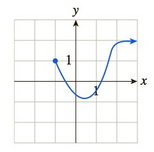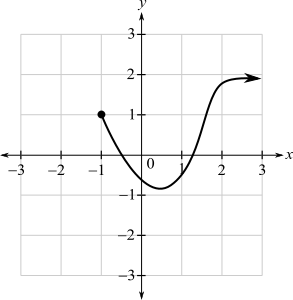Chapter 10.1, Problem 43EFinite Mathematics and Applied Cal...

7th Edition
Stefan Waner + 1 other
ISBN: 9781337274203

Solutions

Chapter
SectionFinite Mathematics and Applied Cal...

7th Edition
Stefan Waner + 1 other
ISBN: 9781337274203
Textbook Problem

In Exercises 35–48 the graph of f is given. Use the graph to compute the quantities asked for. [HINT: See Examples 4–5.]a. lim x → − 1 f ( x ) b. lim x → − 1 f ( x ) c. lim x → − 1 f ( x ) d. f ( − 1 )(a)

To determine

The value of limx1+f(x) by the use of the graph which is as follows:Explanation

Given information:

The graph is as follows:

From the graph, evaluate the limit for x tends to 1+ for the function f(x).

As x is approaching 1 from the right, f(x) appears to approach the solid dot at the height of 1

(b)

To determine

The value of limx1f(x) by the use of the graph which is as follows:(c)

To determine

The value of limx1f(x) by the use of the graph which is as follows:(d)

To determine

The value of f(1) by the use of the graph which is as follows:Still sussing out bartleby?

Check out a sample textbook solution.

See a sample solution

The Solution to Your Study Problems

Bartleby provides explanations to thousands of textbook problems written by our experts, many with advanced degrees!

Get Started

In Exercises 99-106, factor out the greatest common factor from each expression. 99. 4x5 2x4 6x3

Applied Calculus for the Managerial, Life, and Social Sciences: A Brief Approach

Compute C8,8.

Understanding Basic Statistics

In Problems 21-24, if , find each of the following using the properties of logarithms. 22.

Mathematical Applications for the Management, Life, and Social Sciences

Evaluate the integral. 44. 1+exdx

Single Variable Calculus: Early Transcendentals

Finding a Limit In Exercises 11-28, find the limit. lims0(1/1+s)1s

Calculus: Early Transcendental Functions (MindTap Course List)